NCERT Solutions for Class 10 Maths Chapter 14 Statistics Ex 14.2 are part of NCERT Solutions for Class 10 Maths. Here we have given NCERT Solutions for Class 10 Maths Chapter 14 Statistics Ex 14.2.

 Board CBSE Textbook NCERT Class Class 10 Subject Maths Chapter Chapter 14 Chapter Name Statistics Exercise Ex 14.2 Number of Questions Solved 6 Category NCERT Solutions

## NCERT Solutions for Class 10 Maths Chapter 14 Statistics Ex 14.2

Question 1.
The following table shows the ages of the patients admitted in a hospital during a year.Find the mode and the mean of the data given above. Compare and interpret the two measures of central tendency.
Solution:
For Mode: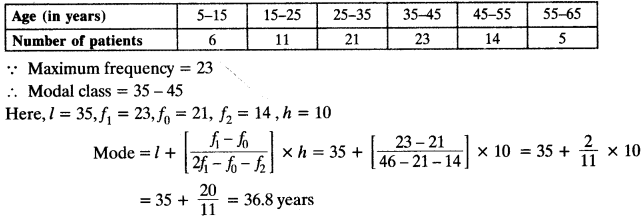For Mean:We conclude that the maximum number of patients in the hospital are of the age 36.8 years. While on an average the age of patient admitted to the hospital is 35.37 years.

Question 2.
The following data gives the information on the observed lifetimes (in hours) of 225 electrical components: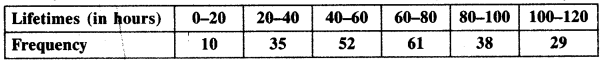Determine the modal lifetimes of the components.
Solution: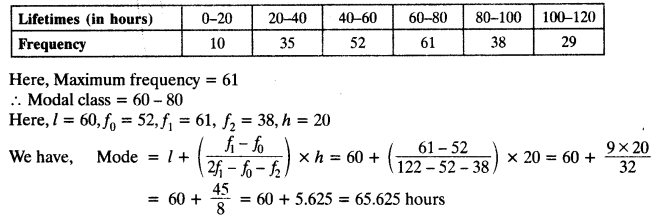Question 3.
The following data gives the distribution of total monthly household expenditure of 200 families of a village. Find the modal monthly expenditure of the families. Also, find the mean monthly expenditure:Solution: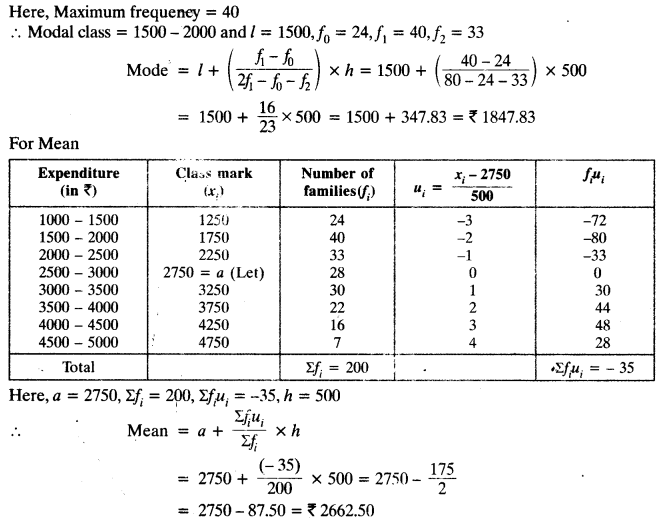Question 4.
The following distribution gives the state-wise teacher- student ratio in higher secondary schools of India. Find the mode and mean of this data. Interpret the two measuresSolution: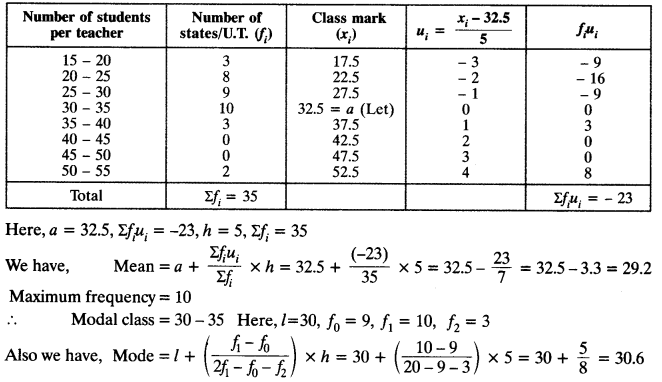Hence, we conclude that most states/U.T. have a student teacher ratio of 30.6 and on an average this ratio is 29.2.

Question 5.
The given distribution shows the number of runs scored by some top batsmen of the world in one – day international cricket matches.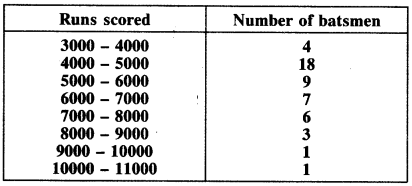Find the mode of the data.
Solution: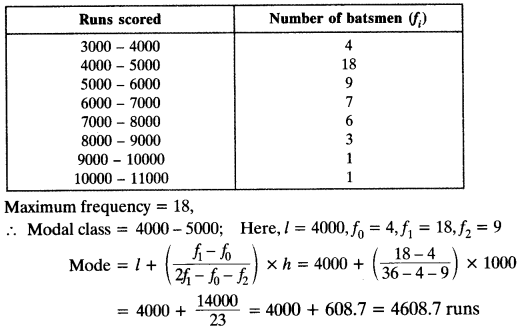Question 6.
A student noted the number of cars passing through a spot on a road for 100 periods each of 3 minutes and summarised it in the table given below. Find the mode of the data:Solution:We hope the NCERT Solutions for Class 10 Maths Chapter 14 Statistics Ex 14.2, help you. If you have any query regarding NCERT Solutions for Class 10 Maths Chapter 14 Statistics Ex 14.2, drop a comment below and we will get back to you at the earliest.